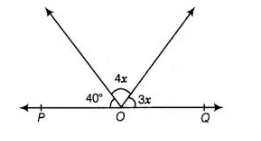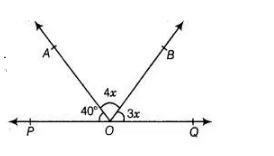# In the figure, POQ is a line.`
Question:

In the figure, POQ is a line. The value of $x$ is(a)20°

(b)25°

(c)30°

(d) 35°

Thinking Process

When two or more rays are initiated from a same point of a line, then the sum of all angles made between the rays and line at the same point is 180°.

Solution:

(a) Since, $P O Q$ is a line segment.

$\therefore \quad \angle P O Q=180^{\circ}$

$\Rightarrow \quad \angle P O A+\angle A O B+\angle B O Q=180^{\circ}$

$\Rightarrow \quad 40^{\circ}+4 x+3 x=180^{\circ}$[putting $\angle P O A=40^{\circ}, \angle A O B=4 x$ and $\angle B O Q=3 x$ ]

$\Rightarrow \quad 7 x=180^{\circ}-40^{\circ}$

$\Rightarrow \quad 7 x=140^{\circ}$

$\Rightarrow \quad x=\frac{140^{\circ}}{7}$

$\therefore \quad x=20^{\circ}$